# SAS/STAT Examples

## Bayesian Multivariate Prior for Multiple Linear Regression

Contents | SAS Program | PDF

## Overview

This example fits a Bayesian multiple linear regression (MLR) model by using a built-in multivariate normal density function MVN in the MCMC procedure for the prior on the regression parameters. By using built-in multivariate distributions, PROC MCMC can efficiently sample constrained multivariate parameters with random walk Metropolis algorithm.

## Analysis

Researchers are interested in determining the relationship of gestational length and litter size on brain weight after accounting for body weight. They study the evalutionary benefits of larger brain weight after accounting for the coinciding disadvantages of longer gestational length and smaller litter size.

The following data set contains the average brain and body weights in grams and kilograms, respectively, the average gestational length in days, and the litter size for 95 different species as given in Sacher and Staffeldt (1974):

```data brainweight;
input brain body gestation litter @@;
log_brain = log(brain);
log_body = log(body);
log_gestation = log(gestation);
datalines;
17.5 3.5 26 1
3.5 0.93 34 4.6
3.15 0.15 46 3
1.14 0.049 51 1.5

... more lines ...

200 39 180 1
210 66 158 1.2
125 49 150 2.4
106 30 151 2
;
```

Figure 1 displays the distribution of each variable. The skewed distributions and the differing orders of magnitude for the minimum and maximum brain weight, body weight, and gestational length suggest the need for the log transformation.

Figure 1 Distributions of Variables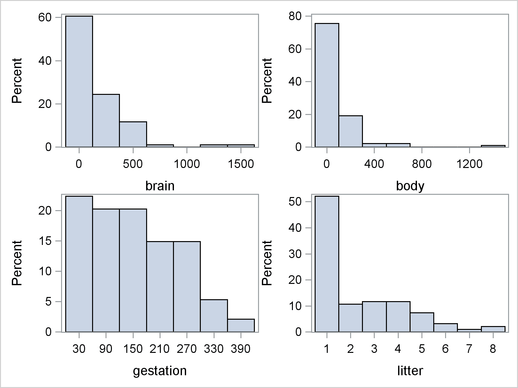## Bayesian MLR Model

Suppose you want to fit a Bayesian MLR model for the logarithm of brain weight with density as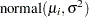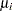(1)

where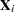is the vector of covariates listed asforspecies. The African elephant has been omitted from this example because it is easily recognized as an extreme outlier even on the log scale.

The likelihood function for the logarithm of the brain weight and corresponding covariates is(2)

where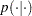denotes a conditional probability density. The normal density is evaluated at the specified value ofand the corresponding mean parameter, which is defined in Equation 1. The four regression parameters in the likelihood arethrough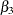.

Suppose you had expert or prior knowledge that some of the covariates were correlated. You might want to use a multivariate prior to incorporate your information. Using the multivariate normal prior, you enable covariates to be independent or correlated a priori. You can also specify the a priori correlation that you believe to be positive or negative. Suppose you thought, a priori, that body weight and gestational age were positively correlated and that body weight and litter size were negatively correlated. Calculate the prior covariance as the product of the prior correlation and standard deviations. More formally, the formula for calculating the covariance of theandcovariate is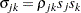where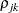is the prior correlation and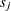and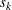are the sample standard deviations of theth andth covariates, respectively.

Suppose the prior correlation between the first and second covariate was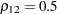, and a priori you thought the standard deviations of the regression coefficients for log of body weight and gestational age wereand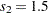, respectively. The prior covariance of the log of body weight and gestational age can be calculated as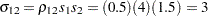. Similarly, suppose the prior correlation between the first and third covariate was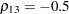and a priori the standard deviation of the litter size was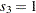. The prior covariance of the log of body weight and litter size can be calculated as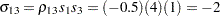.

Suppose the following prior distributions are placed on the parameters: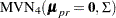(3)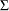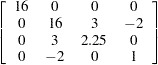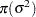(4)

whereindicates a prior distribution,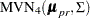indicates a multivariate normal prior of dimension four with mean vectorand variance matrix, and igamma indicates the density function for the inverse-gamma distribution.

The following SAS statements use the likelihood function and prior distributions to fit the Bayesian MLR model with the multivariate prior. The PROC MCMC statement invokes the procedure and specifies the input data set. The NBI= option specifies the number of burn-in iterations. The NMC= option specifies the number of posterior simulation iterations. The THIN=5 option specifies that one of every five samples is saved in the posterior sample. The SEED= option specifies a seed for the random number generator (the seed guarantees the reproducibility of the random stream). The PROPCOV=QUANEW option uses the estimated inverse Hessian matrix as the initial proposal covariance matrix. The MONITOR= option specifies a list of symbols, which can be either parameters or functions of the parameters in the model, for which inference is to be done. The symbol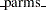is a shorthand for all model parameters, in this case, beta0, beta1, beta2, beta3, sig2. The symbol ExpBeta2 and ExpBeta3 are defined in the program forand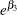, respectively.

```ods graphics on;
proc mcmc data=brainweight nbi=5000 nmc=25000 thin=5 seed=1181
propcov=quanew monitor=(_parms_ ExpBeta2 ExpBeta3);

array data 1 log_body log_gestation litter;
array beta beta0-beta3;
array mu_pr;
array Sigma[4,4];

begincnst;
call zeromatrix(mu_pr);
call identity(sigma);
sigma[1,1]=16;
sigma[2,2]=16;
sigma[3,3]=2.25;
sigma[3,2]=3;
sigma[2,3]=3;
sigma[4,2]=-2;
sigma[2,4]=-2;
endcnst;

parms beta 0;
parms sig2 1;

ExpBeta2=exp(beta2);
ExpBeta3=exp(beta3);

prior beta ~ mvn(mu_pr, Sigma);
prior sig2 ~ igamma(shape = 2.001, scale = 1.001);
call mult(beta, data, mu);
model log_brain~normal(mu,var=sig2);
run;
ods graphics off;
```

The first ARRAY statement declares the data array that stores the covariates of the model. The second ARRAY statement specifies the beta array and the names for the regression coefficients. The next two ARRAY statements declare the hyperparametersand.

The BEGINCNST and ENDCNST statements declare constants in the program. In this call to the MCMC procedure, the CALL to the ZEROMATRIX subroutine fills the prior mean vector with zeros and the IDENTITY subroutine fills in the prior covariance matrix with the identity matrix. The CALL to the MULT subroutine designates 4 on the diagonal instead of 1. The last four statements designate the prior covariances to incorporate your prior correlation.

The first PARMS statement declares regression coefficients to be model parameters and assigns them an initial value of. The second PARM statement declaresas a model parameter and assigns it an initial value of.

ExpBeta1 and ExpBeta2 are the names for the functions of parameters forandthat you want to obtain posterior summary statistics and convergence diagnostics graphs.

The first prior distribution assigns a multivariate normal distribution towith meanand varianceas given in Equation 3. The second PRIOR statement assigns the inverse-gamma prior distribution to theas given in Equation 4.

The CALL statement uses the MULT matrix multiplication subroutine to calculate. The MODEL statement specifies the likelihood function using the normal density function.

Figure 2 displays convergence diagnostic graphs to assess whether the Markov chain has converged. The trace plot indicates that the chain appears to have reached a stationary distribution. The chain also has good mixing and is dense.

Figure 2 Diagnostic Plots for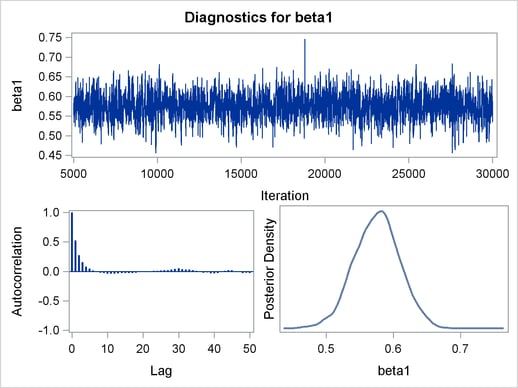The autocorrelation plot indicates low autocorrelation and efficient sampling. Finally, the kernel density plot shows the smooth, unimodal shape of posterior marginal distribution for. In a similar fashion, the rest of the diagnostic plots should be examined to ensure convergence of all parameters.

Figure 3 Posterior Model Summary of Bayesian MLR
The MCMC Procedure

Posterior Summaries
Parameter N Mean Standard
Deviation
Percentiles
25% 50% 75%
beta0 5000 0.9335 0.6698 0.4959 0.9276 1.3798
beta1 5000 0.5755 0.0336 0.5532 0.5762 0.5979
beta2 5000 0.4199 0.1387 0.3268 0.4211 0.5119
beta3 5000 -0.1174 0.0435 -0.1468 -0.1173 -0.0881
sig2 5000 0.2433 0.0357 0.2182 0.2398 0.2652
ExpBeta2 5000 1.5365 0.2141 1.3866 1.5236 1.6685
ExpBeta3 5000 0.8900 0.0387 0.8635 0.8893 0.9156

Posterior Intervals
Parameter Alpha Equal-Tail Interval HPD Interval
beta0 0.050 -0.3815 2.2581 -0.4229 2.1860
beta1 0.050 0.5077 0.6403 0.5113 0.6421
beta2 0.050 0.1470 0.6890 0.1385 0.6770
beta3 0.050 -0.2027 -0.0337 -0.2008 -0.0320
sig2 0.050 0.1831 0.3232 0.1741 0.3115
ExpBeta2 0.050 1.1584 1.9917 1.1335 1.9522
ExpBeta3 0.050 0.8165 0.9669 0.8128 0.9627

Figure 3 reports summary and interval statistics for each regression parameter and the variance parameter. Note that for the slope coefficientboth equal tail interval and HPD interval contain 0.

Figure 4 Diagnostic Plots forand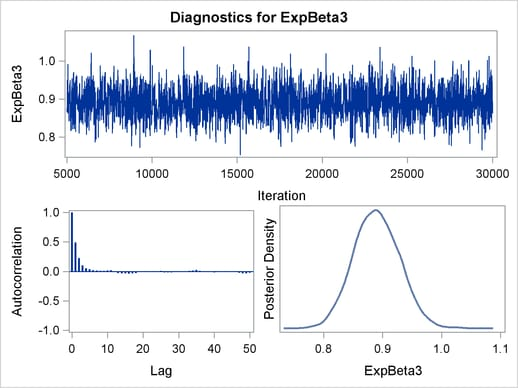Figure 4 displays convergence diagnostic graphs forand. The brain weight increases by a factor of(approximately 54%) for each logarithmic change of one day of gestational age. Similarly, the brain weight decreases by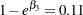(11%) for each addition to the litter size.

## References

Sacher, G. A. and Staffeldt, E. F. (1974), “Relation of Gestation Time to Brain Weight for Placental Mammals: Implications for the Theory of Vertebrate Growth,” The American Naturalist, 108(963), 593–615.